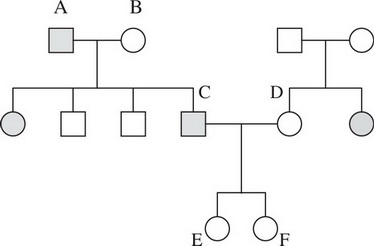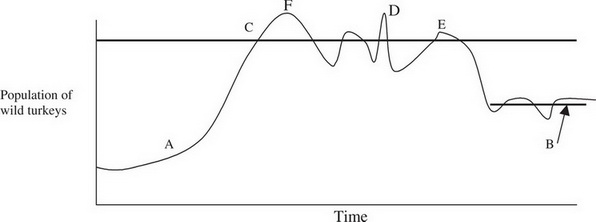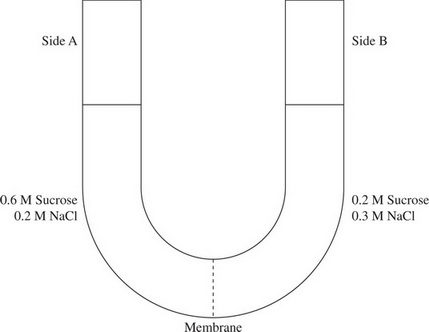# AP Biology Practice Test 26

### Test Information10 questions14 minutes

`Questions 1-3 refer to the following information.An eager AP Biology student interested in studying osmosis and the movement of water in solutions took a dialysis bag containing a 0.5 M solution and placed it into a beaker containing a 0.6 M solution.`

1. After the bag has been sitting in the beaker for a while, what would you expect to have happened to the bag?

2. If this bag were instead placed into a beaker of distilled water, what would be the expected result?

3. Which of the following is true about water potential?

`Questions 4-6 refer to the following information.Questions all use the following pedigree, but are independent of each other:`

4. If the pedigree is studying an autosomal recessive condition for which the alleles are A and a, what was the probability that a child produced by parents A and B would be heterozygous?

5. Imagine that a couple (C and D) go to a genetic counselor because they are interested in having children. They tell the counselor that they have a family history of a certain disorder and they want to know the probability of their firstborn having this condition. What is the probability of the child having the autosomal recessive condition?

6. Imagine that a couple (C and D) have a child (E) that has the autosomal recessive condition being traced by the pedigree. What is the probability that their second child (F) will have the autosomal recessive condition?

`Questions 7-8 refer to the following information.`

7. The bold line that point C intersects is known as the

8. On the basis of what happens at the end of this chart, what is the most likely explanation for the population decline after point E?

`Questions 9-10 refer to the following information.The solutions in the two arms of this U-tube are separated by a membrane that is permeable to water and sodium chloride, but not to sucrose. Side A is filled with a solution of 0.6 M sucrose and 0.2 M sodium chloride (NaCl), and side B is filled with a solution of 0.2 M sucrose and 0.3 M NaCl. Initially, the volume on both sides is the same.`

9. At the beginning of the experiment,

10. If you examine side A after a couple of days, you will see